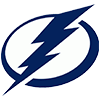DraftKings 2019 Eastern Conference Odds
+400
4 to 1Bruins
16.1% implied probability

+475
4.75 to 1Lightning
14.0% implied probability

+575
5.75 to 1Capitals
11.9% implied probability

+800
8 to 1Hurricanes
8.9% implied probability

+800
8 to 1Islanders
8.9% implied probability

+900
9 to 1Penguins
8.1% implied probability

+900
9 to 1Maple Leafs
8.1% implied probability

+1300
13 to 1Panthers
5.8% implied probability

+1300
13 to 1Flyers
5.8% implied probability

+2000
20 to 1Canadiens
3.8% implied probability

+3000
30 to 1Rangers
2.6% implied probability

+4000
40 to 1Sabres
2.0% implied probability

+5000
50 to 1Blue Jackets
1.6% implied probability

+5500
55 to 1Devils
1.4% implied probability

+10000
100 to 1Senators
0.8% implied probability

+35000
350 to 1Red Wings
0.2% implied probability